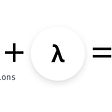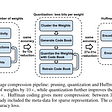# Prerequisites:

This tutorial assumes the following is done:
1. Installation of specific version of CUDA which are supported by tensorrt (cuda 10.2, cuda 11),
2. Install supported CuDNN
3. NVIDIA drivers

# 1. Installation:

On successful CUDA installation, install TensorRT using the following link
https://docs.nvidia.com/deeplearning/tensorrt/install-guide/index.html

To give a gist of the installation, TensorRT can be installed in few ways, out of which I found installing using tar file was the easiest. Installation through debian works but its a bit of a hassle.

Its not necessary to install TensorRT OSS components (https://github.com/NVIDIA/TensorRT)

There are few libraries which turn the model directly to tensorrt:
https://github.com/NVIDIA-AI-IOT/torch2trt
https://github.com/onnx/onnx-tensorrt

But none of it has worked for me. Which is why this tutorial.

This tutorial is to convert onnx model to tensorrt. If you want to know how to convert pytorch model to onnx, you can follow my short tutorial on that

# 2. Convert onnx model to a simplified onnx model

checkout the steps to follow to simplify the onnx model: https://github.com/daquexian/onnx-simplifier

# 3. Convert onnx model to TensorRT engine

`import tensorrt as trtimport pycuda.autoinitimport pycuda.driver as cudadef build_engine(model_file, max_ws=512*1024*1024, fp16=False):    print("building engine")    TRT_LOGGER = trt.Logger(trt.Logger.WARNING)    builder = trt.Builder(TRT_LOGGER)    builder.fp16_mode = fp16    config = builder.create_builder_config()    config.max_workspace_size = max_ws    if fp16:        config.flags |= 1 << int(trt.BuilderFlag.FP16)        explicit_batch = 1 << (int)trt.NetworkDefinitionCreationFlag.\                                                  EXPLICIT_BATCH)    network = builder.create_network(explicit_batch)    with trt.OnnxParser(network, TRT_LOGGER) as parser:        with open(model_file, 'rb') as model:            parsed = parser.parse(model.read())            print("network.num_layers", network.num_layers)            #last_layer = network.get_layer(network.num_layers - 1)            #network.mark_output(last_layer.get_output(0))            engine = builder.build_engine(network, config=config)            return engineengine = build_engine("checkpoints/simplified_model.onnx")`

Save the engine after building it. Because building the engine takes time.

`with open('engine.trt', 'wb') as f:    f.write(bytearray(engine.serialize()))`

# 4. Run Inference:

`runtime = trt.Runtime(TRT_LOGGER)with open('./engine.trt', 'rb') as f:    engine_bytes = f.read()    engine = runtime.deserialize_cuda_engine(engine_bytes)`

Create execution context as shown below

`bert_context = engine.create_execution_context()`

Define inputs as numpy arrays ( input-ids, token-ids and attention-mask ) for BERT.

Define an output variable which also is a numpy array which has shape of batch X num_of_classes. If your model is not BERT, then define a zeros array of shape same as your model output.

`'''inputs'''input_ids = numpy array ( size: batch X seq_len) ex: (1 X 30 )token_type_ids = numpy array ( size: batch X seq_len) ex: (1 X 30 )attention_mask = numpy array ( size: batch X seq_len) ex: (1 X 30 )'''outputs'''bert_output = torch.zeros((1, num_of_classes),device=device).cpu().\                                                   detach().numpy()`

Allocate memory for the inputs and outputs in GPU:

`'''memory allocation for inputs'''d_input_ids = cuda.mem_alloc(batch_size * input_ids.nbytes)d_token_type_ids = cuda.mem_alloc(batch_size * token_type_ids.\                                                       nbytes)d_attention_mask = cuda.mem_alloc(batch_size * attention_mask.\                                                       nbytes)'''memory allocation for outputs'''d_output = cuda.mem_alloc(batch_size * bert_output.nbytes)`

Create bindings array

`bindings = [int(d_input_ids), int(d_token_type_ids), int(d_attention_mask), int(d_output)]`

Create stream and transfer inputs to GPU (can be sync or async ). ‘async ’ shown here.

`stream = cuda.Stream()# Transfer input data from python buffers to device(GPU)cuda.memcpy_htod_async(d_input_ids, input_ids, stream)cuda.memcpy_htod_async(d_token_type_ids, token_type_ids, stream)cuda.memcpy_htod_async(d_attention_mask, attention_mask, stream)`

Execute using the engine

`bert_context.execute_async(batch_size, bindings, stream.handle, None)`

Transfer output back from GPU to python buffer variable

`cuda.memcpy_dtoh_async(bert_output, d_output, stream)stream.synchronize()`

Now the bert_output variable in which we stored zeros will have the prediction.

Run softmax and get the most probable class

`pred = torch.tensor(bert_output)pred_output_softmax = nn.Softmax()(pred)_, predicted = torch.max(pred_output_softmax, 1)`

The effort to convert feels worthwhile when the inference time is drastically reduced.

onnxruntime( GPU ): 0.67 sec
pytorch( GPU ): 0.87 sec
pytorch( CPU ): 2.71 sec
ngraph( CPU backend ): 2.49 sec with simplified onnx graph
TensorRT : 0.022 sec

which is 40x inference speed :) compared to pytorch model

Hope this helps :)
I apologize if I have left out any references from which I could have taken the code snippets from.

# References:

--

--

--

Lazy, Infrequent blogger

Love podcasts or audiobooks? Learn on the go with our new app.

## Building Apps With Microservices: The How & Why## How to Continuously Deploy a CRA Using GitHub Actions and Zeit## Running Ansible from Jenkins in CentOS## Finding broken access controls through source code in .NET applications## Python for Data Science and Machine Learning: An Introduction## What is a CDN, I want to know## Hemanth Sharma

Lazy, Infrequent blogger

## How to Train a Sparse Neural Radiance Field, on AWS EC 2## How to Train a Scalable Classifier with FastAPI and SerpApi ?## Primer on Pytorch’s Dataset class## Quantization in Deep Neural Networks